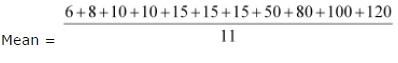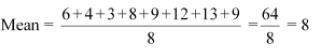# NCERT Solutions class 7 Maths Chapter-3 Exercise 3.2

NCERT Solutions Class-7 Maths chapter 3data handlingExercise-3.2 is prepared by academic team of Physics Wallah all the questions of NCERT text book are solved step by step with proper and detail solutions explaining each and every questions. For More and additional questions ofCBSE class 7 mathyou can go to class 7 maths sections.NCERT class 7 Maths Solutionsis the best way to enhanced your mathematics skill. And Physics Wallah practice worksheet & question bank will help you a lot.

## Question 1:

The scores in mathematics test (out of 25) of 15 students is as follows:

19, 25, 23, 20, 9, 20, 15, 10, 5, 16, 25, 20, 24, 12, 20

Find the mode and median of this data. Are they same?

Scores of 15 students in mathematics test are

19, 25, 23, 20, 9, 20, 15, 10, 5, 16, 25, 20, 24, 12, 20

Arranging these scores in an ascending order,

5, 9, 10, 12, 15, 16, 19, 20, 20, 20, 20, 23, 24, 25, 25

Mode of a given data is that value of observation which occurs for the most number of times. Median of a given data is the middle observation when the data is arranged in an ascending or descending order.

As there are 15 terms in the given data, therefore, the median of this data will be the 8th observation.

Hence, median = 20

Also, it can be observed that 20 occurs 4 times (i.e., maximum number of times).

Therefore, mode of this data = 20

Yes, both are same.

Question 2:

The run scored in a cricket match by 11 players is as follows:

6, 15, 120, 50, 100, 80, 10, 15, 8, 10, 15

Find the mean, mode and median of this data. Are the three same?

The runs scored by 11 players are

6, 15, 120, 50, 100, 80, 10, 15, 8, 10, 15

Arranging these scores in an ascending order,

6, 8, 10, 10, 15, 15, 15, 50, 80, 100, 120Mode of a given data is that value of observation which occurs for the most number of times and the median of the given data is the middle observation when the data is arranged in an ascending or descending order.

As there are 11 terms in the given data, therefore, the median of this data will be the 6th observation.

Median = 15

Also, it can be observed that 15 occurs 3 times (i.e., maximum number of times).

Therefore, mode of this data = 15

No, these three are not same.

Question 3:

The weights (in kg.) of 15 students of a class are:

38, 42, 35, 37, 45, 50, 32, 43, 43, 40, 36, 38, 43, 38, 47

(i) Find the mode and median of this data.

(ii) Is there more than one mode?

The weights of 15 students are

38, 42, 35, 37, 45, 50, 32, 43, 43, 40, 36, 38, 43, 38, 47

Arranging these weights in ascending order,

32, 35, 36, 37, 38, 38, 38, 40, 42, 43, 43, 43, 45, 47, 50

(i)Mode of a given data is that value of observation which occurs for the most number of times and the median of the given data is the middle observation when the data is arranged in an ascending or descending order.

As there are 15 terms in the given data, therefore, the median of this data will be the 8th observation.

Hence, median = 40

Also, it can be observed that 38 and 43 both occur 3 times (i.e., maximum number of times).

Therefore, mode of this data = 38 and 43

(ii)Yes, there are 2 modes for the given data.

Question 4:

Find the mode and median of the data: 13, 16, 12, 14, 19, 12, 14, 13, 14

The given data is

13, 16, 12, 14, 19, 12, 14, 13, 14

Arranging the given data in an ascending order,

12, 12, 13, 13, 14, 14, 14, 16, 19

Mode of a given data is that value of observation which occurs for the most number of times and the median of the given data is the middle observation when the data is arranged in an ascending or descending order.

As there are 9 terms in the given data, therefore, the median of this data will be the 5th observation.

Hence, median = 14

Also, it can be observed that 14 occurs 3 times (i.e., maximum number of times).

Therefore, mode of this data = 14

Question 5:

Tell whether the statement is true or false:

(i) The mode is always one of the numbers in a data.

(ii) The mean is one of the numbers in a data.

(iii) The median is always one of the numbers in a data.

(iv) The data 6, 4, 3, 8, 9, 12, 13, 9 has mean 9.

(i) True

The given statement is true.

Since,

We know that,

Mode is termed as that value of observation which occurs for the maximum number of times.

Hence,

Mode of a given data is that value of observation which occurs for the most number of times. Therefore, it is one of the observations given in the data.

(ii) False

Mean may or may not be one of the numbers in the data.

(iii) True

The given statement is true.

Since,

We know that,

Median of any data is known as the middle observation when that data is arranged in ascending or descending order.

Hence,

Median is always one of the numbers in a data

Therefore,

The median of the given data is the middle observation when the data is arranged in an ascending or descending order.

(iv) False

The given data is 6, 4, 3, 8, 9, 12, 13, 9